# Division - 7th grade (12y) - math problems

#### Number of problems found: 243

• Bottles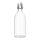The weight of 17 bottles is 91.7 kg. What is the weight of 1 bottle?
• RemainderWhat is the remainder of the division of natural numbers 293 and 7?
• Coke and coal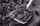20.1 tons of coke is produced from 30 tons of black coal. How much coke is made from 1 kilogram of coal?
• Wooden blockA cuboid-shaped wooden block has 6 cm length, 4 cm breadth, and 1 cm height. Two faces measuring 4 cm x 1 cm are colored in black. Two faces measuring 6 cm x 1 cm are colored in red. Two faces measuring 6 cm x 4 cm are colored in green. The block is divid
• Dividing walnuts into crates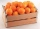There are 8 and 2 over 3 pounds of walnuts in a container, which will be divided equally into containers that hold 1 and 1 over 5 pounds. This would fill n and 4 over 18 containers. What is n?
• PegsFrom two sticks 240 cm and 210 cm long, it is necessary to cut the longest possible pegs for flowers so that no residues remain. How many pegs will it be?
• The excavation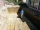The excavation for the city sewer was 38 m long, 2.2 m wide, and 3 m deep. a) How many cubic meters of soil were dredged b) How many journeys had to be made by one car when removing the soil, if they loaded an average of 4.5 m of cubic soil on each journe
• Three ships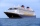There are three ships moored in the port, which sail together. The first ship returns after two weeks, the second after four weeks, and the third after eight weeks. In how many weeks the ships will meet in the port for the first time? How many times have
• Grandma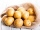6 kg of potatoes is enough for four at home for 15 days. For how many days will this amount be enough if a grandma will visit?
• Thousand ballsWe have to create a thousand balls from a sphere with a diameter of 1 m. What will be their radius?
• CannonballsOf the three cannonballs with a diameter of 16 cm, which landed in the castle courtyard during the battle, the castle blacksmith cast balls with a diameter of 10 cm, which fit into the cannons placed on the walls. How many cannonballs did the blacksmith c
• Divisible by nineHow many three-digit natural numbers in total are divisible without a remainder by the number 9?
• Father and daughterFather is 36 years old, daughter is 20 years less. What will be the ratio between them when they are 10 years more?
• A section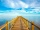A section of the boardwalk is made of 15 boards. Each board is 9 1/4 inches wide. The total width of the boardwalk is 144 inches. The spacing between each board is equal. What is the width of the spacing between the boards?
• Result and remainderAfter dividing the unknown number by the number 23, the quotient 11 and the remainder 4 are formed. Find an unknown number.
• Identical cubesFrom the smallest number of identical cubes whose edge length is expressed by a natural number, can we build a block with dimensions 12dm x 16dm x 20dm?
• Into boxHow many cubes with an edge of 2.5 cm fit into a box measuring 11.6 cm; 8.9 cm and 13.75 cm?
• Square gardensThe gardening colony with dimensions of 180 m and 300 m is to be completely divided into equally large square areas with the largest possible area. Calculate how many such square areas can be obtained and determine the side length of the square.
• Video gameNicole is playing a video game where each round lasts 7/12 of an hour. She has scheduled 3 3/4 hours to play the game. How many rounds can Nicole play?
• Chicks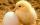How many chicks were hatched from 4500 eggs, when an average of 100 eggs hatched 87 chicks?

Do you have an interesting mathematical word problem that you can't solve it? Submit a math problem, and we can try to solve it.

We will send a solution to your e-mail address. Solved examples are also published here. Please enter the e-mail correctly and check whether you don't have a full mailbox.

Please do not submit problems from current active competitions such as Mathematical Olympiad, correspondence seminars etc...